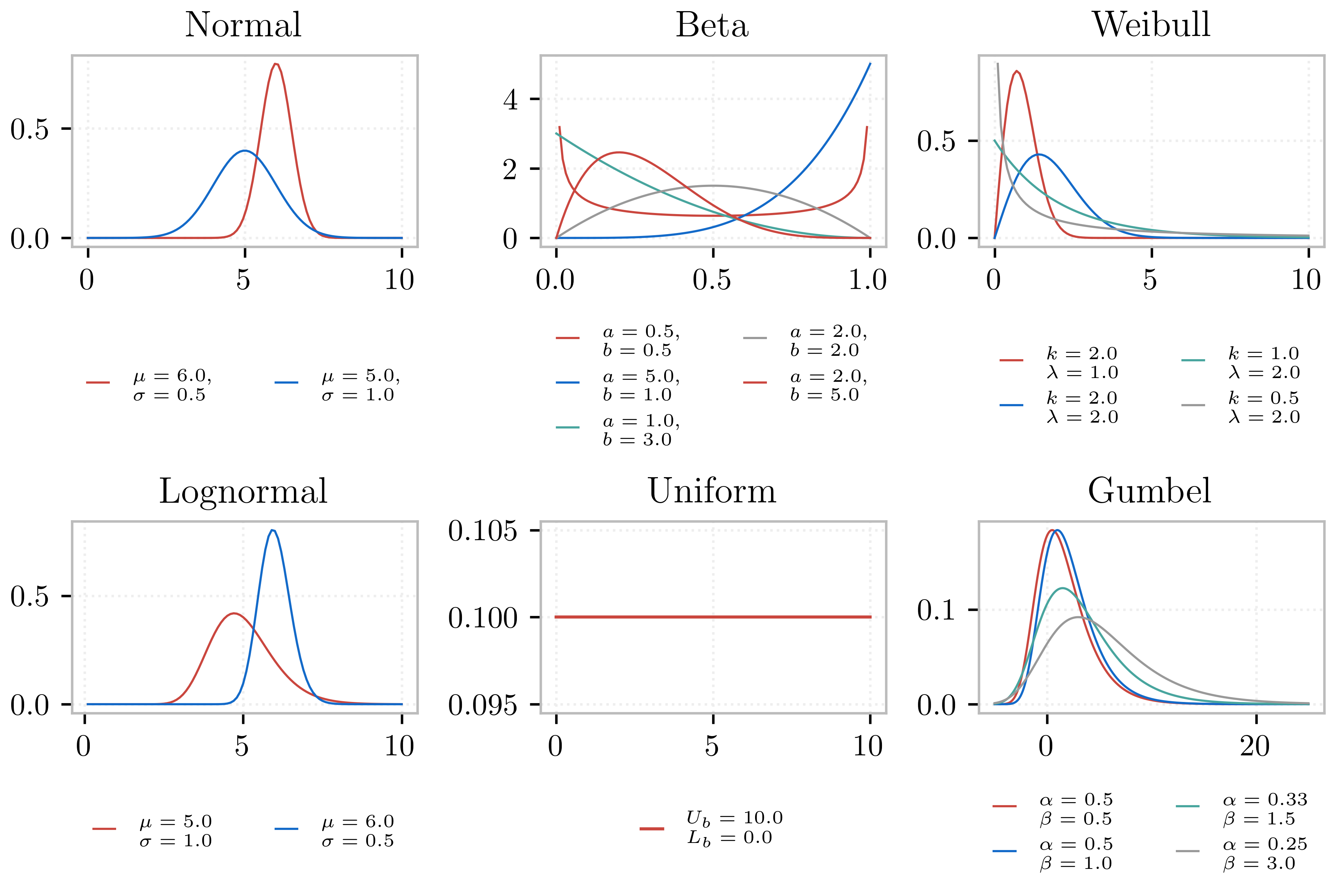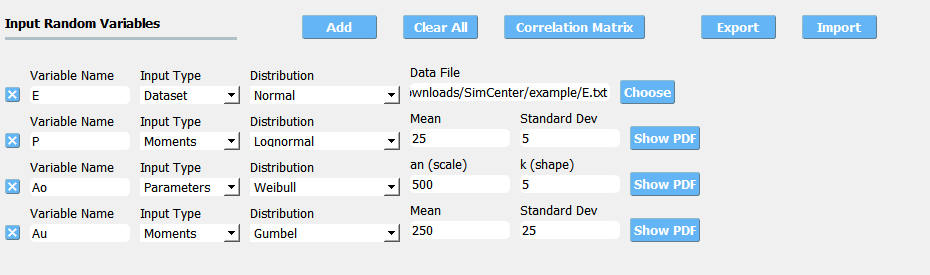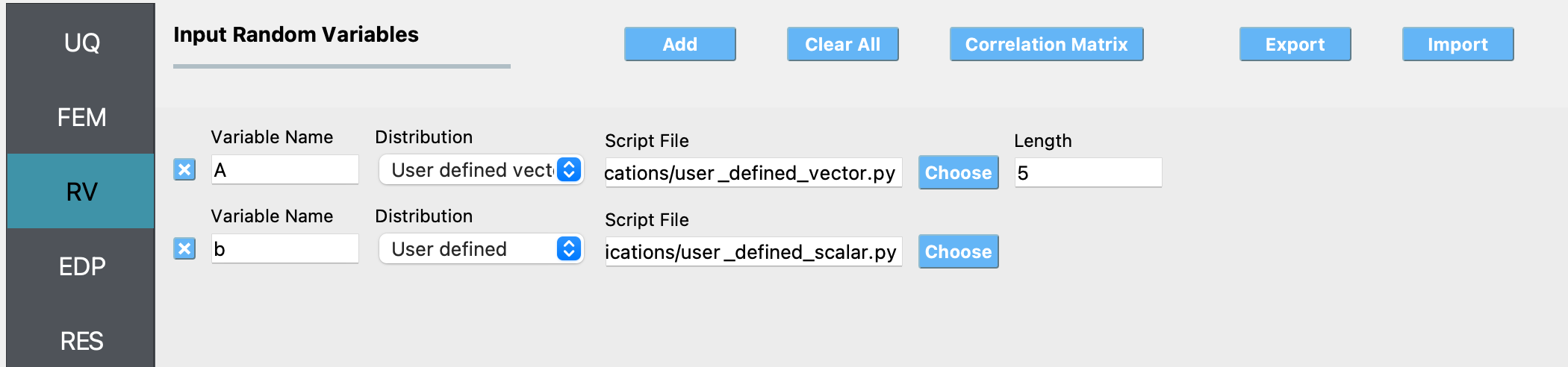# 3.3. RV: Random Variables¶

The RV tab allows the user to specify the probabilistic distribution for the random problem at hand. The following probabilistic distributions for the random variables are currently supported:

## 3.3.1. Probability distribution¶

Note

To add a new random variable the user presses the Add button. To remove a random variable, the user must first select it by checking the small circle before the random variable, and then pressing the Remove button.

### 3.3.1.1. Dakota Engine¶

The following six distribution classes are supported for Dakota engine.

1. Normal (Gaussian)

User provides the mean ($$\mu$$) and standard deviation ($$\sigma$$) of the normal distribution. The density function of the normal distribution, as a function of $$\mu$$ and $$\sigma$$ is:

(3.3.1.1.1)$f(x) = \frac{1}{\sqrt{2 \pi} \sigma} \exp \left( -{\frac{1}{2} \left( \frac{x - \mu}{\sigma} \right)^2} \right)$
2. Lognormal

User provides the mean ($$\mu$$) and standard deviation ($$\sigma$$) of the lognormal distribution. The density function of the lognormal distribution, as a function of $$\mu$$ and $$\sigma$$ is:

(3.3.1.1.2)$f(x) = \frac{1}{\sqrt{2 \pi} \zeta x} \exp \left( -{\frac{1}{2} \left( \frac{\ln x - \lambda}{\zeta} \right)^2} \right)$

where $$\zeta^2 = \ln \left( \frac{\sigma^2}{\mu^2} + 1 \right)$$ and $$\lambda = \ln(\mu) - \frac{\zeta^2}{2}$$

1. Beta

User provides $$\alpha$$, $$\beta$$, lower bound ($$L_B$$), and an upper bound ($$U_B$$) for the beta distribution. The density function of the normal distribution, as a function of these quantities is:

(3.3.1.1.3)$f(x) = \frac{\Gamma(\alpha + \beta)}{\Gamma(\alpha)\Gamma(\beta)} \frac{(x - L_B)^{\alpha-1}(U_B-x)^{\beta-1}}{(U_B - L_B)^{\alpha + \beta - 1}}$

where $$\Gamma(\alpha)$$ is the Gamma function.

2. Uniform

User provides the lower bound ($$L_B$$), and an upper bound ($$U_B$$) for the uniform distribution. The density function of the normal distribution, as a function of these quantities is:

(3.3.1.1.4)$f(x) = \frac{1.0}{(U_B - L_B)}$

The mean of the distribution is $$\frac{(U_B + L_B)}{2.0}$$

3. Weibull

User provides shape parameter ($$k$$) and scale parameter ($$\lambda$$) for the Weibull distribution. The density function of the Weibull distribution, as a function of these quantities is:

(3.3.1.1.5)$f(x) = \frac{k}{\lambda}\left(\frac{x}{\lambda}\right)^{k-1} \exp \left( -(x/\lambda)^{k} \right)$

where $$k,\lambda > 0$$ and $$x \geq 0$$. For $$x<0$$, $$f(x) = 0$$.

4. Gumbel

User provides $$\alpha$$ and $$\beta$$ for the Gumbel distribution, where $$\beta$$ is known as the location parameter and $$\frac{1}{\alpha}$$ the scale parameter. The density function of the Gumbel distribution, as a function of these quantities is:

(3.3.1.1.6)$f(x) = \alpha e^{-\alpha(x-\beta)} \exp(-e^{-\alpha(x-\beta)})$

For each random variable, the user must enter a name and select from the pull down menu the distribution associated with the random variable. For the distribution selected, the user must then provide the input arguments, which are as described above. Fig. 3.3.1.1.1 shows the panel for a problem with four Random Variables with all random input following Gaussian distributions.Fig. 3.3.1.1.1 Random variable specification.

Note

To add a new random variable the user presses the Add button. To remove a random variable, the user must first select it by checking the small circle before the random variable, and then pressing the Remove button.

Warning

Removing a random variable may have unintended consequences and cause the UQ engine to fail.Fig. 3.3.1.1.2 Various distributions offered by the quoFEM app .

### 3.3.1.2. SimCenterUQ Engine¶

Five additional distributions are supported in the SimCenter UQ engine. The users can define distributions either by Parameters, Moments or Dataset. (Note: Nataf transform module developed by [ERA19] is adopted)

1. Exponential

User provides the parameter ($$\lambda$$) of the exponential distribution. The density function of the exponential distribution, as a function of $$\lambda$$ is:

(3.3.1.2.1)$f(x) = \lambda \exp(-\lambda x)$

where $$x>0$$ and $$\lambda>0$$. User can alternatively provide the mean ($$m$$) of the exponential distribution.

(3.3.1.2.2)$m = \frac{1}{\lambda}$
2. Discrete

User provides the $$N$$ discrete values ($$x_i$$) and their weights (probability $$p_i$$) for a multinomial distribution. The probability mass function of the discrete distribution is:

(3.3.1.2.3)$\begin{split}p(x)=\begin{cases} p_i, & \text{if x=x_i}\\ 0, & \text{otherwise} \end{cases}\end{split}$

where $$p_i>0$$. The weights ($$p_i$$) will be automatically normalized if they do not sum up to one. The option to define by moments is not supported for the discrete distribution.

3. Gamma

User provides the shape parameter ($$k$$) and scale parameter ($$\lambda$$) of the Gamma distribution. The density function of the Gamma distribution, as a function of $$k$$ and $$\lambda$$ is:

(3.3.1.2.4)$f(x) = \frac{\lambda^kx^{k-1}\exp(-\lambda x)}{\Gamma(k)}$

where $$\lambda>0$$ and $$k>0$$. User can alternatively provide the mean ($$m$$) and standard deviation ($$\sigma$$) .

(3.3.1.2.5)$\begin{split}m &= \frac{k}{\lambda} \\ \sigma &= \sqrt{\frac{k}{\lambda^2}}\end{split}$
4. Chi-squared

User provides the parameter $$k$$ of the Chi-squared distribution. The density function of the Chi-squared distribution, as a function of $$k$$ is

(3.3.1.2.6)$f(x) = \frac{1}{2^{\frac{k}{2}}\Gamma\left(\frac{k}{2}\right)}x^{\left(\frac{k}{2}-1\right)} \exp\left(-\frac{x}{2}\right)$

where $$x>0$$ and $$k$$ is a natural number. User can alternatively select the moment option where the mean ($$m$$) is

(3.3.1.2.7)$m = k$
5. Truncated exponential

User provides the parameter $$k$$ and bounds $$L_B$$ and $$U_B$$ for the truncated exponential distribution. The density function of the truncated exponential distribution, as a function is

(3.3.1.2.8)$f(x) = \frac{\lambda}{c} \exp(-\lambda x), \text{ where L_B<x<U_B}$

where $$c$$ is a normalization constant, i.e.

(3.3.1.2.9)$c = \int_{L_B}^{U_B} \lambda\exp(-\lambda x) dx$

where $$x>0$$ and $$\lambda>0$$. User can alternatively provide the mean of the distribution along with the truncated bounds.Fig. 3.3.1.2.1 Extended random variable specification

Input Type - Dataset

User can also define the random variables by providing sample realization data set as shown in the below figure, by selecting Dataset input type. The data will be fitted to the specified probability distribution model. Note that for some of the bounded distributions, such as beta and truncated exponential, the bounds should additionally be provided through the user interface.Fig. 3.3.1.2.2 Example of input dataset file

Note

• Clicking the Show PDF or Show PMF button will display probability distribution (or mass) function of each random variable with the specified parameters/moments. If the PDF or PMF is not displayed, we recommend the users to double-check the parameters/moments if they are in a valid range. The plotting button is not activated for the Dataset input type.

ERA19

Engineering Risk Analysis Group, Technische Universität München: https://www.bgu.tum.de/era/software/eradist/ (Matlab/python programs and documentations)

### 3.3.1.3. UCSD-UQ Engine¶

The same set of distributions that are supported by the SimCenterUQ engine are supported in the UCSD-UQ engine. Currently, the distributions can only be defined through their Parameters. Correlation between the random variables is not supported in the UCSD-UQ engine.

### 3.3.1.4. CustomUQ Engine¶

The same set of distributions and correlation options as that supported by the SimCenterUQ engine can also be selected when the CustomUQ engine is used. Additionally, two options - User defined and User defined vector are also available.

When the User defined option is chosen, the user must provide the path to a script file that makes the desired functionality for the CustomUQ engine available, such as methods to draw samples from the user-defined distribution, or to evaluate the probability density of the user-defined distribution at specified sample points, etc.

When the User defined vector option is chosen, in addition to the path to the script file, the user must also enter the number of components in the random vector.Fig. 3.3.1.4.1 Specifying user-defined distributions under CustomUQ engine

## 3.3.2. Correlation matrix¶

Correlation coefficients between each variable can be defined by clicking the Correlation Matrix button. Default correlations between variables are set to be zero. The diagonal element of the matrix is fixed as one, and symmetry of the correlation matrix is enforced once the entries of the lower triangular part of the matrix are modified.Fig. 3.3.2.1 Example of a valid correlation matrix

Once the OK button is clicked after setting all required entries, the program will automatically check the validity of the matrix before closing the correlation matrix window. If the matrix is not positive definite, an error message will be displayed and the window will not be closed. In such case, user should adjust the correlation coefficients to be positive definite.

Note

• When constant variable is introduced instead of probability distributions, the correlation coefficient corresponding to those variables will be ignored.

• When more than one random variable is provided as Dataset, correlations between the data pairs will not be incorporated automatically. If correlations exist, user can define them manually at the correlation matrix window.

Warning

Correlation warping for Nataf variable transformation of beta distributions is not currently supported.